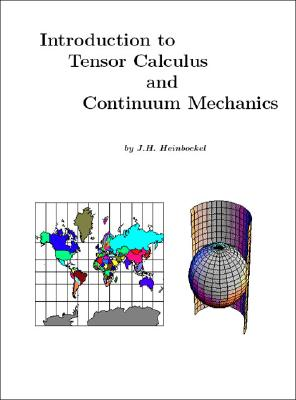### Introduction to Tensor Calculus and Continuum Mechanics

Paperback

List Price: 42.50*
* Individual store prices may vary.

#### Description

Introduction to Tensor Calculus and Continuum Mechanics is an advanced College level mathematics text. The first part of the text introduces basic concepts, notations and operations associated with the subject area of tensor calculus. The material presented is developed at a slow pace with a detailed explanation of the many tensor operations. The first half of the text concludes with an introduction to the application of tensor concepts to differential geometry and relativity. The second half of the text presents applications of tensors to areas from continuum mechanics. Tensor calculus is applied to the areas of dynamics, elasticity, fluids, electricity and magnetism. Many of the basic equations from physics, engineering and science are developed which makes the text an excellent reference work. The second half of the text concludes with an introduction to quaternions, multivectors and Clifford algebra.

There are four Appendices. The Appendix A contains units of measurements from the Syst me International d'Unit's along with some selected physical con stants. The Appendix B contains a listing of Christoffel symbols of the second kind associated with various coordinate systems. The Appendix C is a summary of use ful vector identities. The Appendix D contains solutions to selected exercises. The text has numerous illustrative worked examples and over 450 exercises.

Trafford Publishing, 9781553691334, 432pp.

Publication Date: August 23, 2006